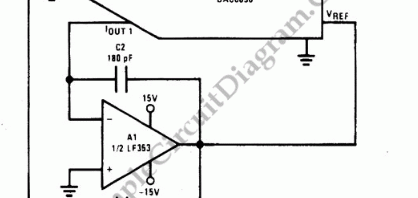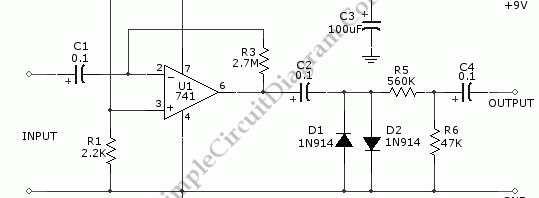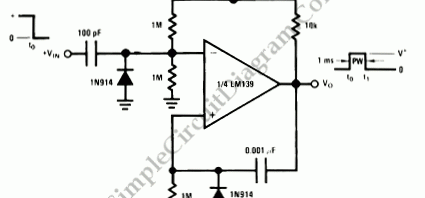## Voltage to Frequency Converter Using UJTThe following voltage to frequency converter (V/FC – VCO) circuit consist of a UJT (uni-junction transistor) oscillator in which the timing charge capacitor C2 is linearly depends on the input signal voltage. The voltage across resistor R5 set the charging current, which is accurately controlled by the amplifier. Here is the schematic diagram of the circuit:

## Programmable Capacitance Multiplier with DACCapacitance multiplier simulate high capacitance capacitor for analog signal signal processing. By using DAC (Digital-to-Analog Converter), we can simulate variable capacitance with very wide range, which can be used in digitally controlled analog processor (programmable filters for example). The following circuit shows a DAC0830 connected as a capacitance multiplier. This circuit is a DAC controlled amplifier (used capacitance multiplication) to […]

## Fuzz Box Distortion Pedal – Guitar EffectThis guitar effect circuit uses a simple high gain amplification, followed by a symmetric clipping using parallel diodes clipper. The gain of the 741 op-amp pre amplifier will be R3/(total impedance of guitar pickup + C1).  With 1 kOhm guitar pickup and C1 combination impedance, the gain would be more than 1000x, this would be enough to make sure the […]